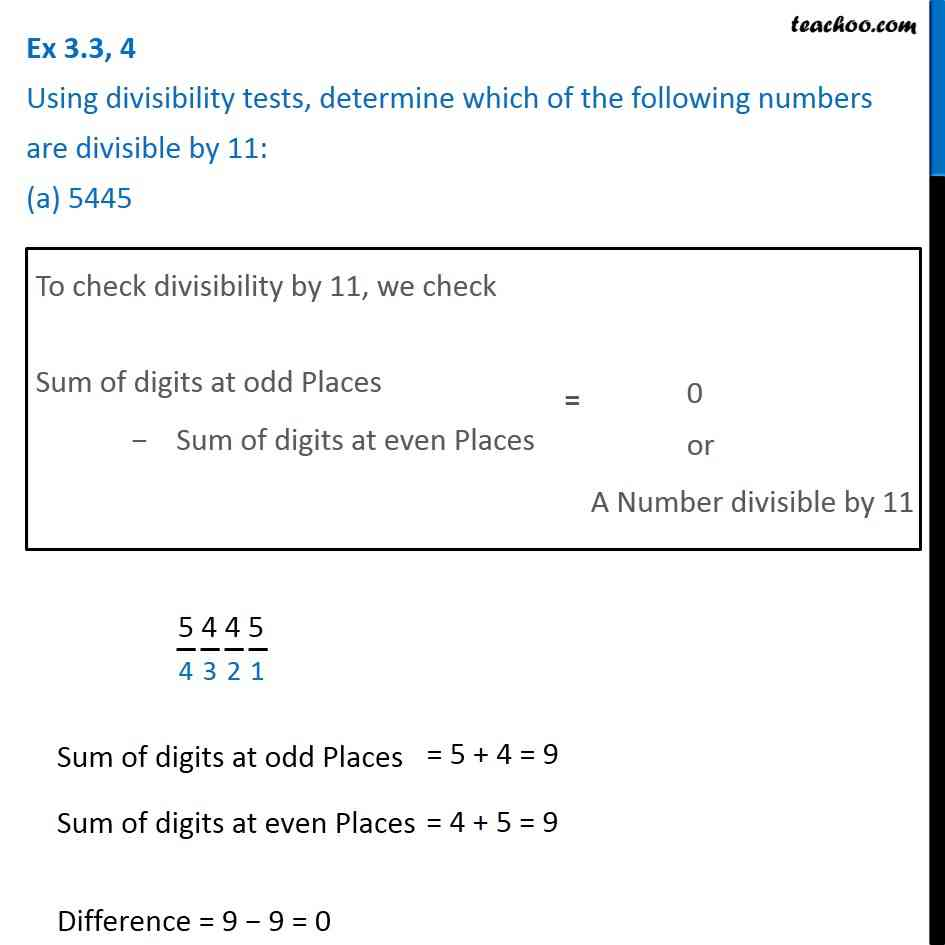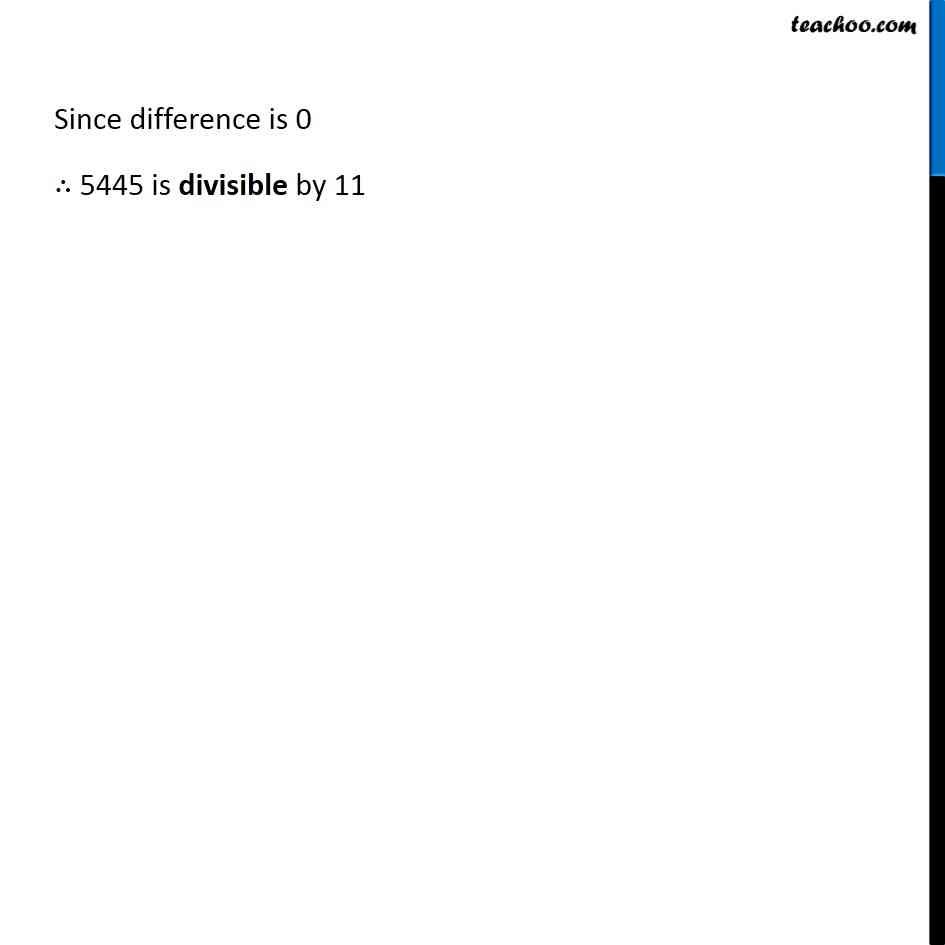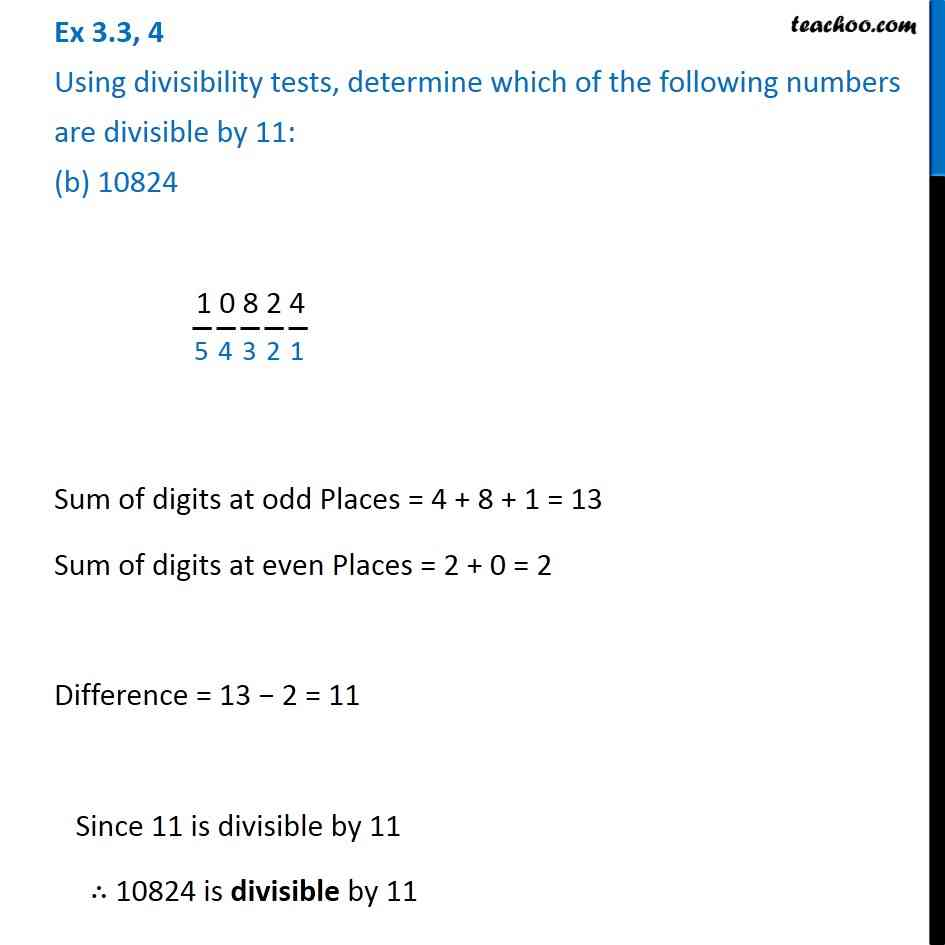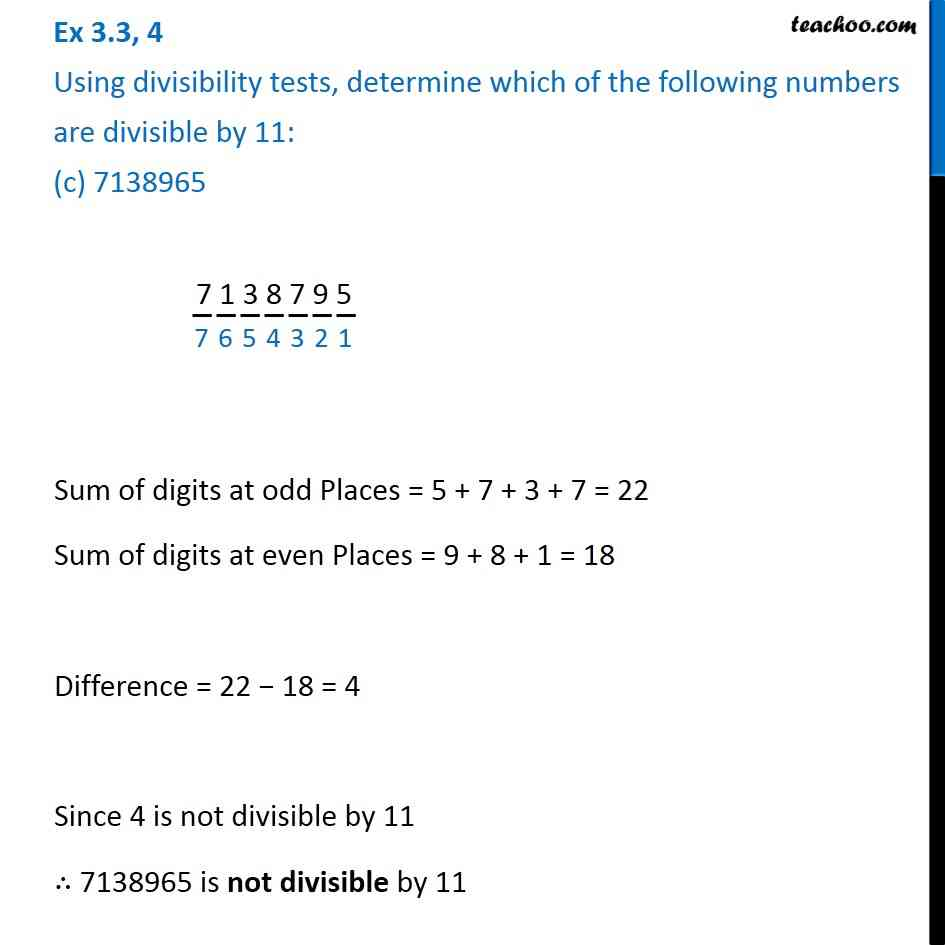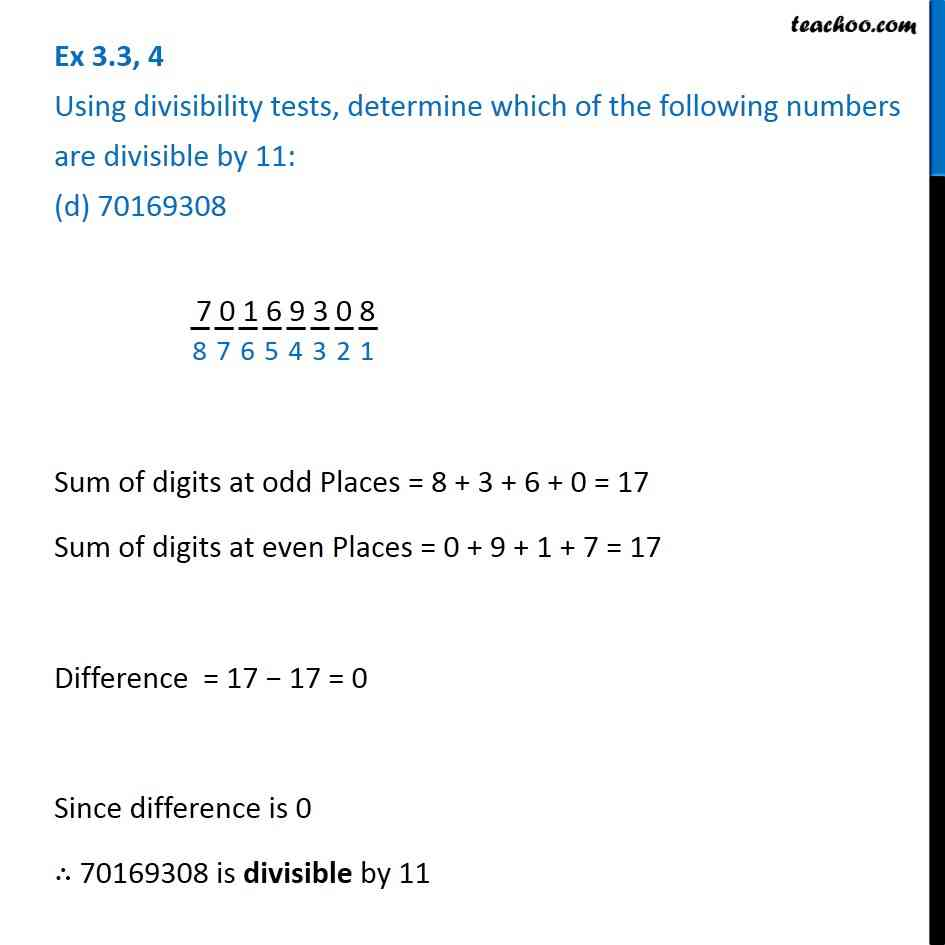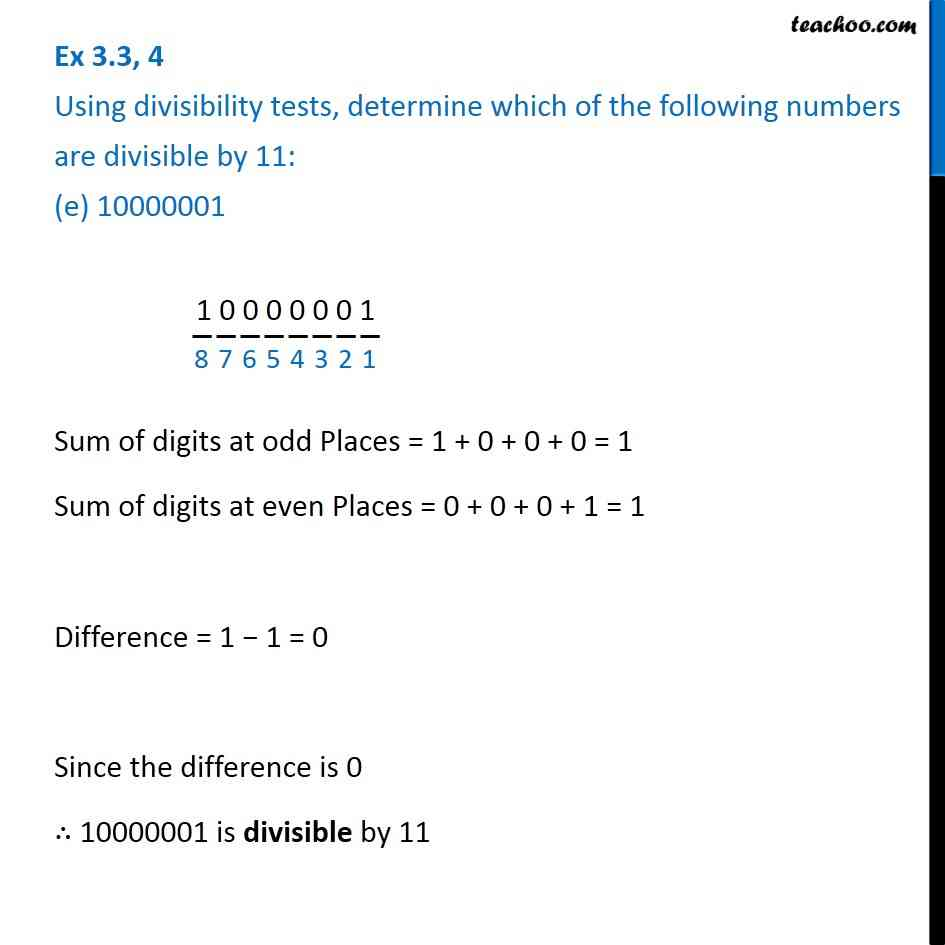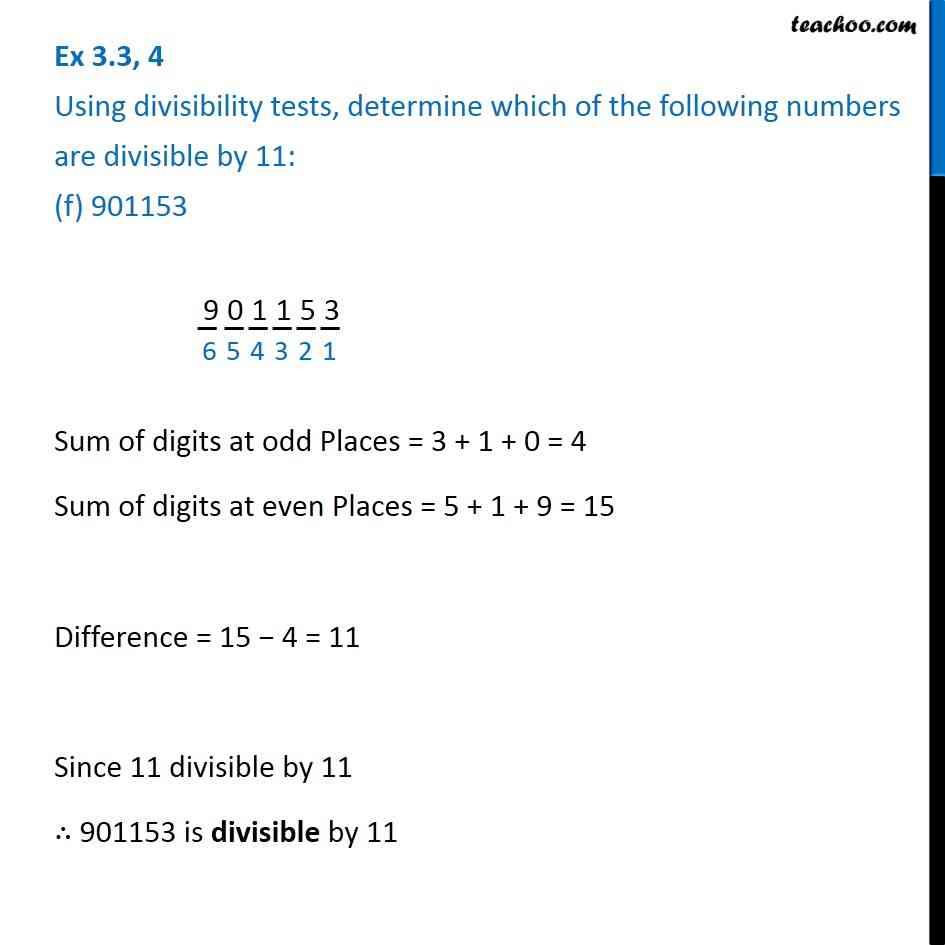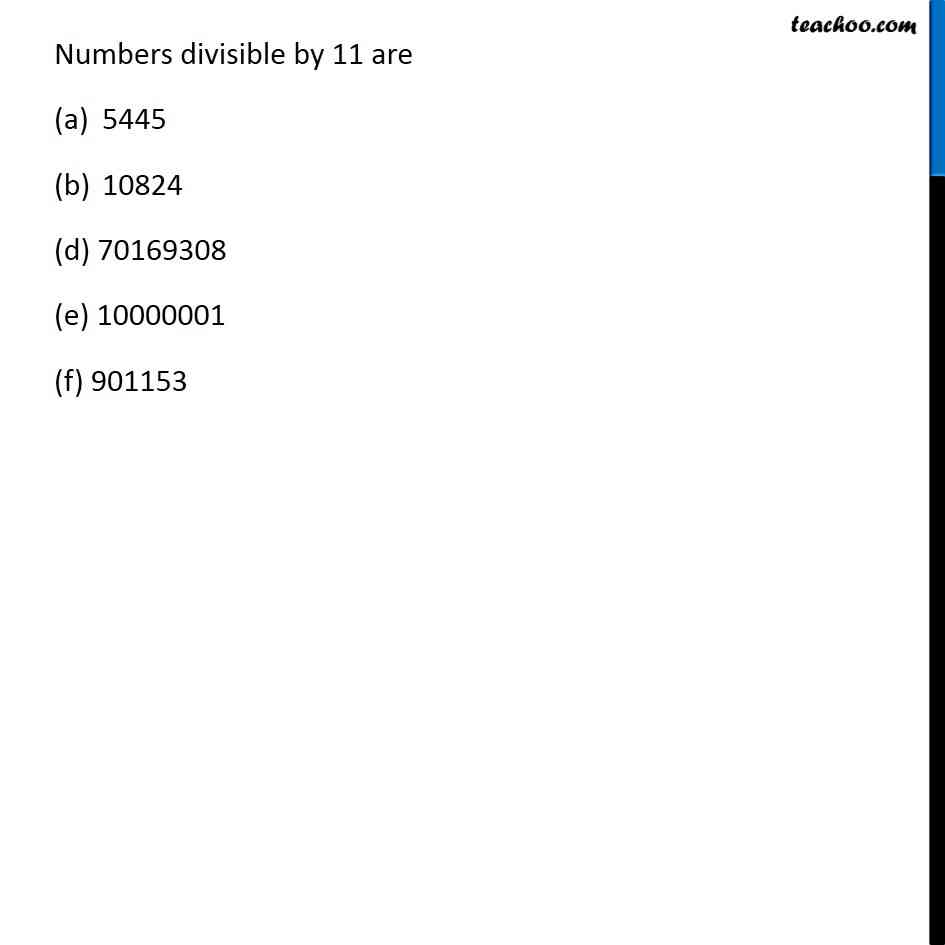1. Chapter 3 Class 6 Playing with Numbers
2. Serial order wise
3. Ex 3.3

Transcript

Ex 3.3, 4 Using divisibility tests, determine which of the following numbers are divisible by 11: (a) 5445To check divisibility by 11, we check Sum of digits at odd Places − Sum of digits at even Places 5 4 4 5 Sum of digits at odd Places Sum of digits at even Places Difference = 9 − 9 = 0 Since difference is 0 ∴ 5445 is divisible by 11 Ex 3.3, 4 Using divisibility tests, determine which of the following numbers are divisible by 11: (b) 10824 1 0 8 2 4 Sum of digits at odd Places = 4 + 8 + 1 = 13 Sum of digits at even Places = 2 + 0 = 2 Difference = 13 − 2 = 11 Since 11 is divisible by 11 ∴ 10824 is divisible by 11 Ex 3.3, 4 Using divisibility tests, determine which of the following numbers are divisible by 11: (c) 7138965 7 1 3 8 7 9 5 Sum of digits at odd Places = 5 + 7 + 3 + 7 = 22 Sum of digits at even Places = 9 + 8 + 1 = 18 Difference = 22 − 18 = 4 Since 4 is not divisible by 11 ∴ 7138965 is not divisible by 11 Ex 3.3, 4 Using divisibility tests, determine which of the following numbers are divisible by 11: (d) 70169308 7 0 1 6 9 3 0 8 Sum of digits at odd Places = 8 + 3 + 6 + 0 = 17 Sum of digits at even Places = 0 + 9 + 1 + 7 = 17 Difference = 17 − 17 = 0 Since difference is 0 ∴ 70169308 is divisible by 11 Ex 3.3, 4 Using divisibility tests, determine which of the following numbers are divisible by 11: (e) 10000001 1 0 0 0 0 0 0 1 Sum of digits at odd Places = 1 + 0 + 0 + 0 = 1 Sum of digits at even Places = 0 + 0 + 0 + 1 = 1 Difference = 1 − 1 = 0 Since the difference is 0 ∴ 10000001 is divisible by 11 Ex 3.3, 4 Using divisibility tests, determine which of the following numbers are divisible by 11: (f) 901153 901153 Sum of digits at odd Places = 3 + 1 + 0 = 4 Sum of digits at even Places = 5 + 1 + 9 = 15 Difference = 15 − 4 = 11 Since 11 divisible by 11 ∴ 901153 is divisible by 11 Numbers divisible by 11 are 5445 10824 (d) 70169308 (e) 10000001 (f) 901153

Ex 3.3

About the AuthorDavneet Singh
Davneet Singh is a graduate from Indian Institute of Technology, Kanpur. He has been teaching from the past 10 years. He provides courses for Maths and Science at Teachoo.